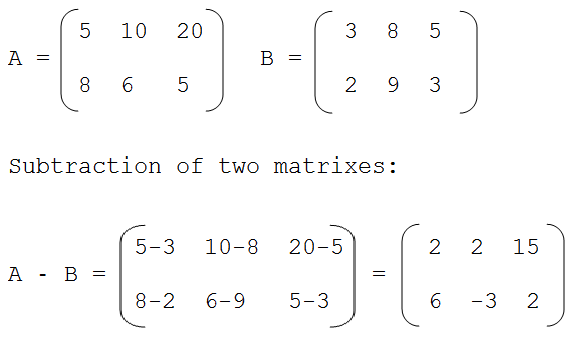# Java Program To Subtract Two Matrices – 3 Ways

Java program for matrix subtraction. The following Java code to subtract two matrices has been written in three different ways. For addition you can check here, The methods are:

• Using For Loop
• Using While Loop
• Using Do-While Loop

The compiler has been added to the program so that you can execute and check it for yourself, along with suitable examples and sample outputs.

Matrix subtraction is carried out between two matrices of the same order. A detailed example is shown as below:Source

## Using For Loop

1) If two matrices have same size then only we can subtract the two matrices.

2) Read row number “row”,column number “col” using scanner class method.

3) Insert  elements at mat1[i][j] using two for loops with the structures for ( i=0;i <row;i++ ),for (j= 0 ; j<col;i++ ) , similarly insert elements at mat2[i][j].i indicates row number and j indicates column number.

4) For subtraction of two matrices

• for i=0 to row-1.
• for j=0 to col-1.
• mat1[i][j] – mat2[i][j] and insert the result at res[i][j].

5) Print res[][] matrix to display the subtraction of two matrices.

Output:

### Using While Loop

1) To insert elements at the matrix

• while loop iterates until i<row
• while loop iterates until j<col
• insert the element at mat1[i][j],
• j value increased by 1.
• i value increased by 1

2) Similarly insert elements at mat2[][].

3)For subtraction of two matrices

• while loop iterates until i<row.
• inner while loop iterates until j<col.
• subtract mat1[i][j] – mat2[i][j]  and insert in to the matrix at res[i][j].
• increase the j value by 1.
• increase the i value.

4) Print the two-dimensional array res[][] to display the subtraction of two matrices.

Output:

#### Using Do-While Loop

Output:

More Java Programs:

x

## Plus Star Pattern Java Program | Patterns

Java program to print plus star pattern program – We have written the below print/draw ...